# 教程1

## 要求：

1. 一部支持NFC的手机
2. 知道IC卡的密钥ab

（不知道的请下载M keys进行尝试破解）

## 步骤：

### 第二步(添加规则)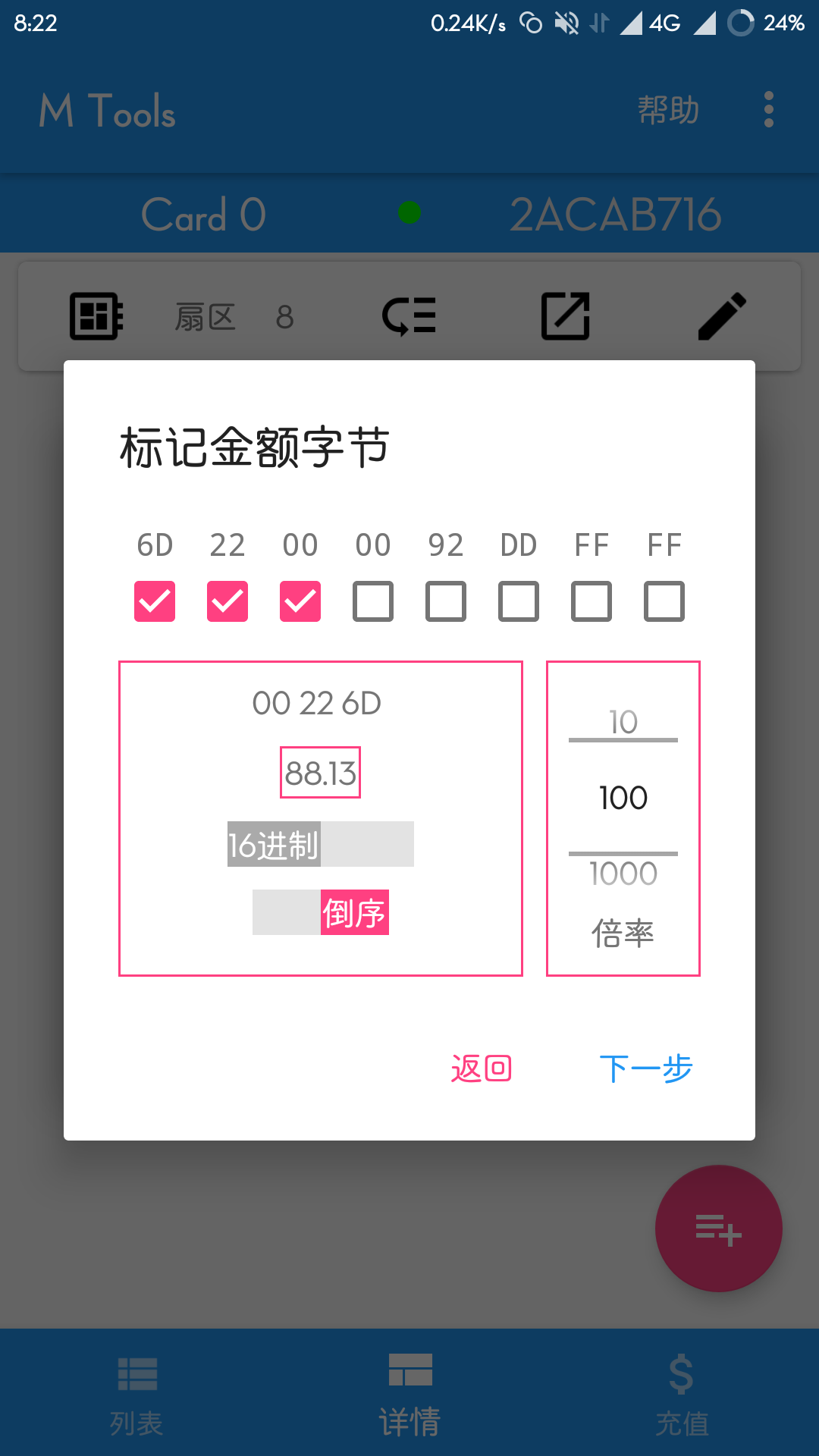### 第三步(标记校验字节)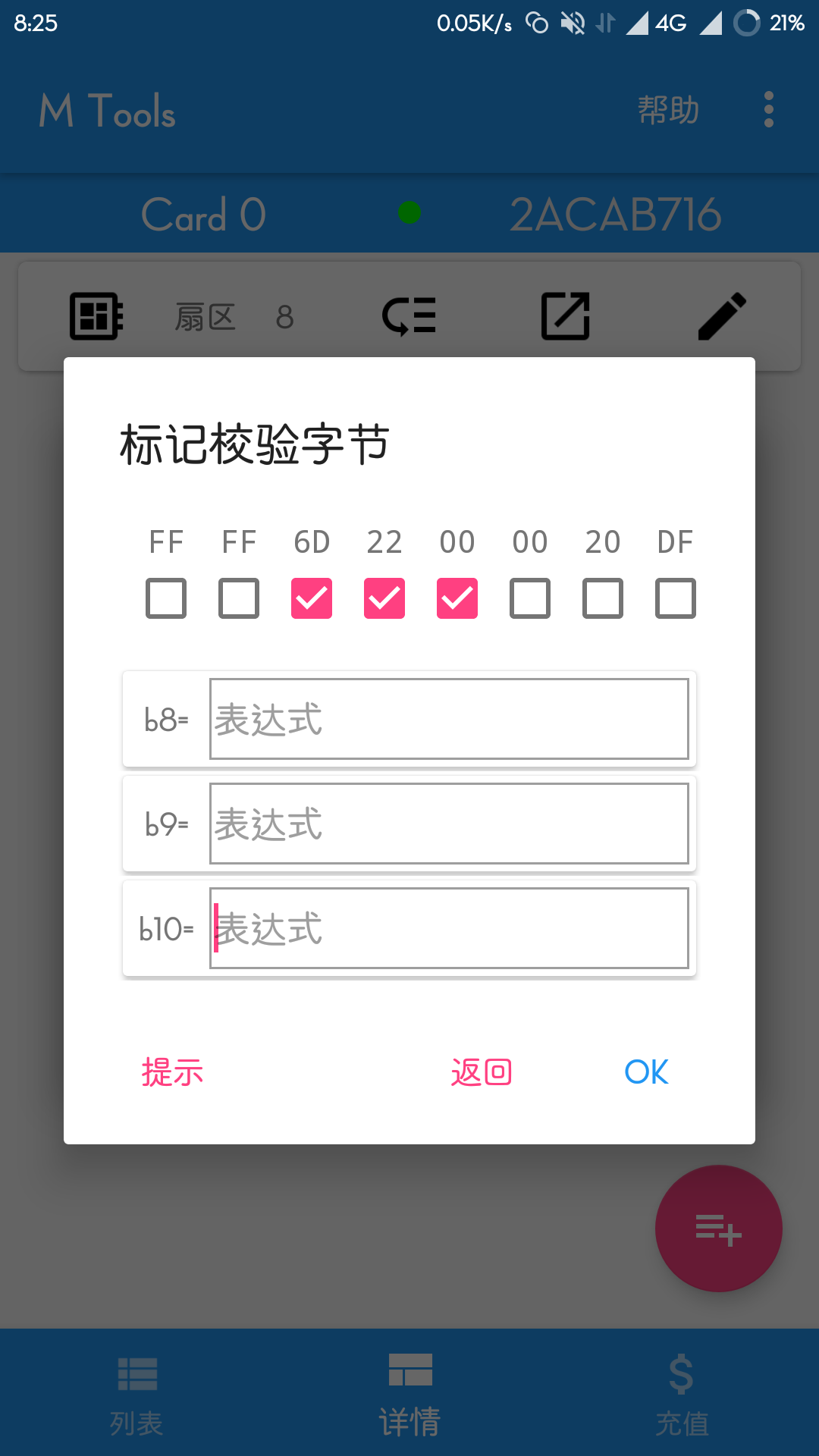``````
6D 20 00 00 FF FF FF FF 6D 20 00 00 20 DF 20 DF
``````

``````
b8=(b0)

b9=(b1)

b10=(b2)
``````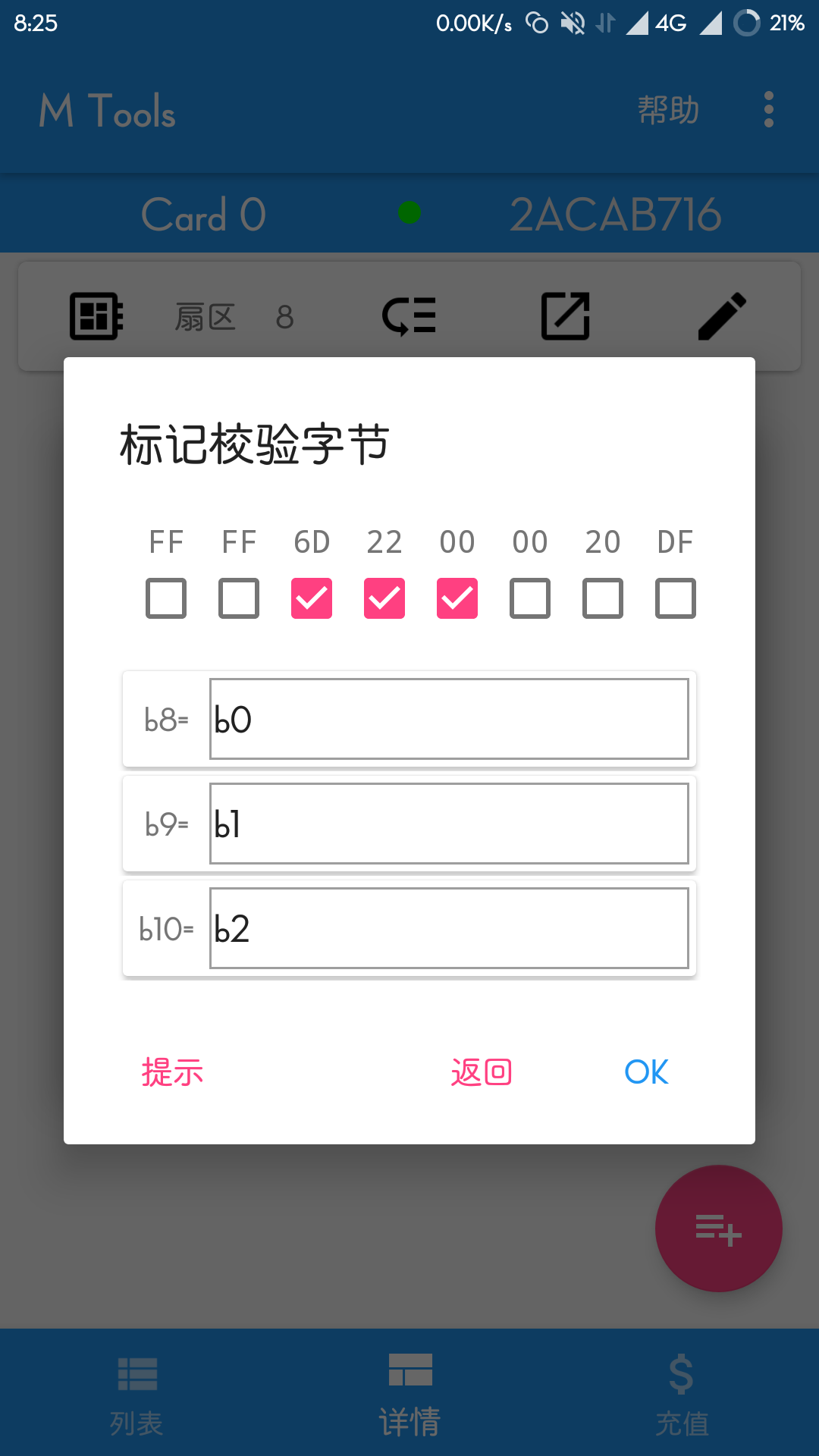``````
b2=b1 →b2=b1
b4=b2+0x1F → b4=b2+31
b15=b0 xor b1 → [email protected]^b1
b2=not b0 → [email protected]~b0
``````

### 第四步(充值金额)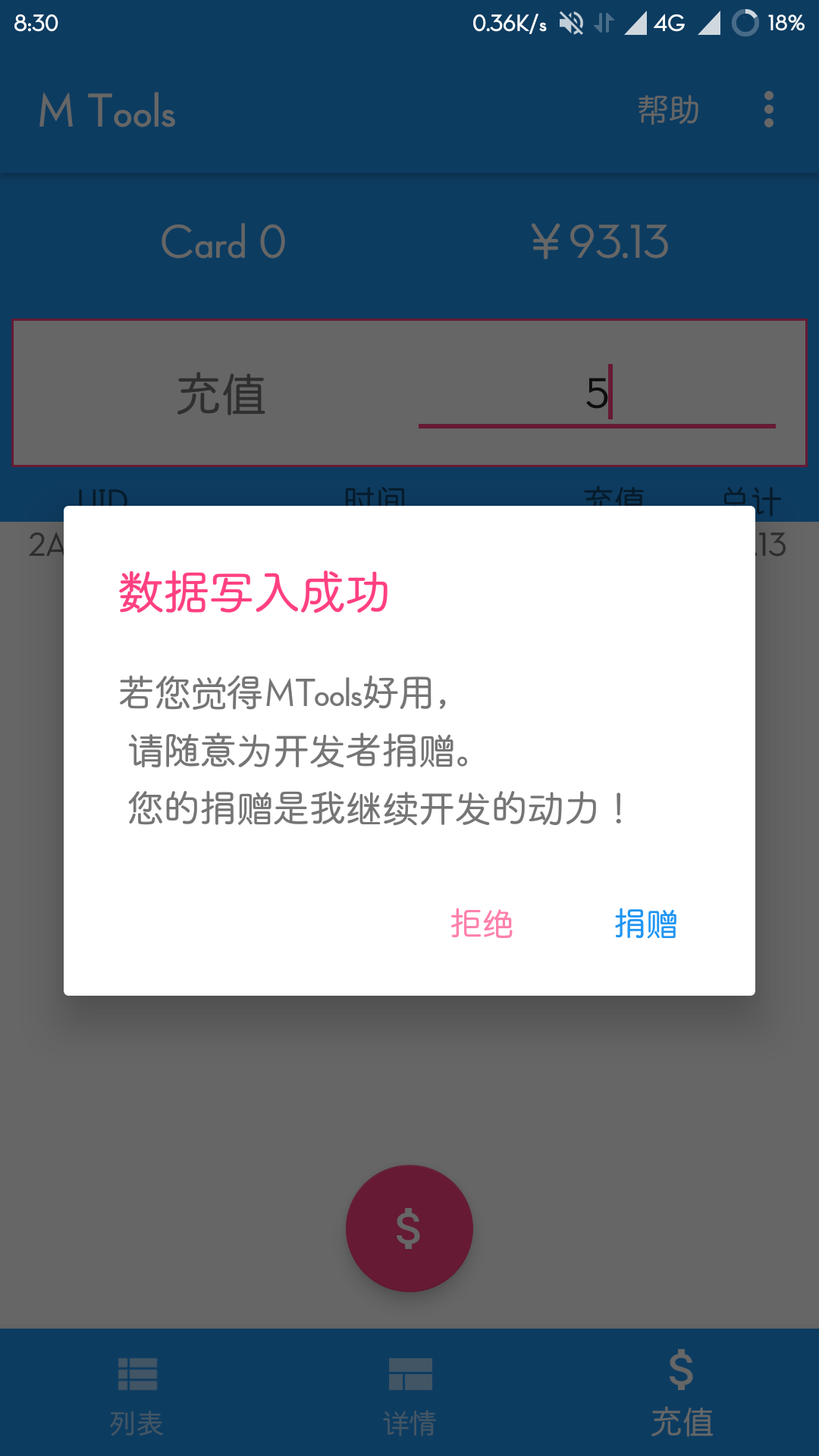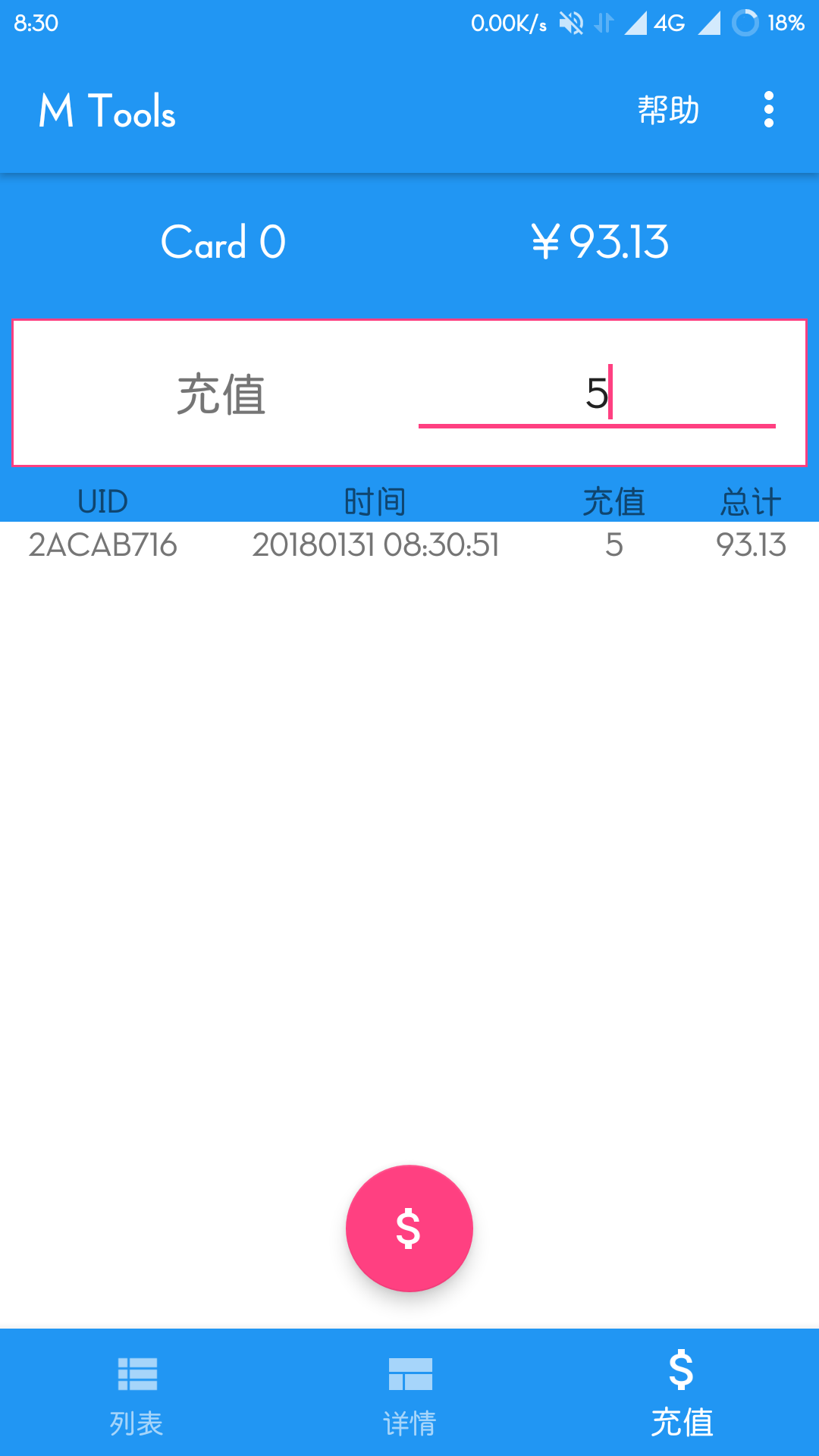ps:如果出错请检查你的算法及表达式